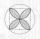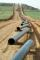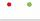# Square

If we increase side of the square, increase its area of 63%.
What is the percentage we increase side of the square?

Result

p =  27.7 %

#### Solution:Leave us a comment of example and its solution (i.e. if it is still somewhat unclear...):

Showing 0 comments:Be the first to comment!#### To solve this example are needed these knowledge from mathematics:

Our percentage calculator will help you quickly calculate various typical tasks with percentages. Do you want to convert length units?

## Next similar examples:

1. QuatrefoilCalculate area of the quatrefoil which is inscribed in a square with side 6 cm.
2. Content area and percentsDetermine what percentage is smaller cube surface, when the surface area of the wall decreases by 25%.
3. PipelineHow much percent has changed (reduced) area of pipe cross-section, if circular shape changed to square with same perimeter?
4. Diameters of circlesHow many percent of the area of a larger circle is a smaller circle if the smaller circle has a diameter 120 mm and a larger one has a diameter 300 mm?
5. ClassIn 7.C clss are 10 girls and 20 boys. Yesterday was missing 20% of girls and 50% boys. What percentage of students missing?
6. A perineumA perineum string is 10% shorter than its original string. The first string is 24, what is the 9th string or term?
7. Green - RedWe have 5 bags. Each consist one green and 2 red balls. From each we pull just one ball. What is the probability that we doesn't pull any green ball?
8. Map 3Map scale is M = 1: 25000 . Two cottages which are shown on the map are actually 15 km away. What is its distance on the map?
9. Theorem proveWe want to prove the sentense: If the natural number n is divisible by six, then n is divisible by three. From what assumption we started?
10. Simple interest 3Find the simple interest if 11928 USD at 2% for 10 weeks.
11. Simple interest 4Find the simple interest if 5243 USD at 4.3% for 261 days. Assume a 361-day year.
12. IronIron ore contains 57% iron. How much ore is needed to produce 20 tons of iron?
13. Scale of the mapDetermine the scale of the map if the actual distance between A and B is 720 km and distance on the map is 20 cm.
14. Geometric progression 2There is geometric sequence with a1=5.7 and quotient q=-2.5. Calculate a17.
15. SequenceBetween numbers 1 and 53 insert n members of the arithmetic sequence that its sum is 702.
16. Complex number coordinatesWhich coordinates show the location of -2+3i
17. Calculation of CNCalculate: ?